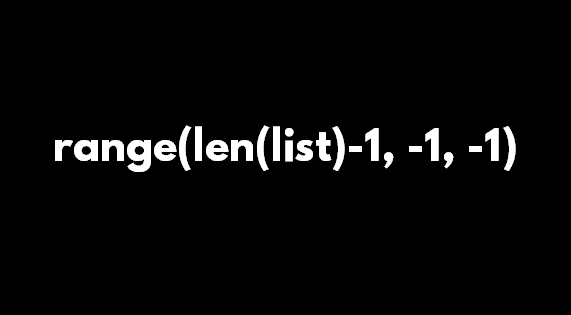×

Search anything:

# Range len-1 in Python

#### PythonGet this book -> Problems on Array: For Interviews and Competitive Programming

In this article, we have explained the Python code snippet "range(len(list)-1, -1, -1)" and other variants of the code snippet.

In short, the for loop in Python "for i in range(len(list)-1, -1, -1)" is used to loop through all elements of a list in reverse order that is from end to first.

``````for i in range(len(list)-1, -1, -1):
# do something like learning at OpenGenus
``````

We will start with a basic for loop in Python and incrementally change the code to arrive to Range len-1 in Python.1. Range in Python
2. range(len(list))
3. range(len(list)-1)
4. range(len(list)-1, 0, -1)
5. range(len(list)-1, -1, -1)

## Range in Python

Range is a built-in function in Python that is used to generate a list of numbers. The structure of range is as follows:

``````range(starting_index, last_index, increment)
``````
• starting_index: This is optional and the default value is 0.
• last_index: This is the value at which the iteration or list need to be stopped. This is a complusory parameter.
• increment: This is the value by which the starting index is incremented at each step. This is optional and the default value is 1.

This generates a list of numbers starting with the number "starting_index", incrementing with value "increment" and ending before the number "last_index".

If range(2, 6, 1), then the sequence is:
2, 3, 4, 5

If range(9, 15, 2), then the sequence is:
9, 11, 13

## range(len(list))

As only the ending index is complusory, then if one number is provided to range() then the starting number is set to 0 and increment is set to 1.

range(len(list)) is used to generate all sequence of indices in a list as the ending number is length of the list and the starting index is 0 so that last index is length-1.

Consider the following Python code snippet:

``````list = [10, 5, 99, -90, 12]
for i in range(len(list)):
print("Index ", i, ": ", list[i])
print()
``````

Following will be the output of the above Python code:

``````Index  0 :  10
Index  1 :  5
Index  2 :  99
Index  3 :  -90
Index  4 :  12
``````

## range(len(list)-1)

In this variant, we have updated len(list) to len(list)-1 that is we are eliminating the last element of the list.

Consider the following Python code snippet:

``````list = [10, 5, 99, -90, 12]
for i in range(len(list)-1):
print("Index ", i, ": ", list[i])
print()
``````

Following will be the output of the above Python code:

``````Index  0 :  10
Index  1 :  5
Index  2 :  99
Index  3 :  -90
``````

Note the last element at index 4 which is 12 is not printed.

## range(len(list)-1, 0, -1)

In this variant, we have provided all parameters. The starting index is the last index of the list and sequence will terminate when 0 is generated. The increment is -1 that is the sequence is decremented by 1 at each step.

This will generate the indices of the list in reverse order but not as the index starts from 0 and the sequence will end when 0 is generated, the first index of the list will not be generated.

Consider the following Python code snippet:

``````list = [10, 5, 99, -90, 12]
for i in range(len(list)-1, 0, -1):
print("Index ", i, ": ", list[i])
print()
``````

Following will be the output of the above Python code:

``````Index  4 :  12
Index  3 :  -90
Index  2 :  99
Index  1 :  5
``````

Note the first element that is at index 0 which is 10 is not printed.

## range(len(list)-1, -1, -1)

This is the final variant where there are multiple -1s. As explained by the syntax, the starting element is len(list)-1 that is the last index of the list.

-1 is the increment factor so this will decrement the values by 1 at each step.

The sequence will stop when -1 is generated so the last element will be 0 which is the first index of the list.

Hence, this will generate the list of indices of list in reverse order. This can be used to print a list in reverse order.

Consider the following Python code snippet:

``````list = [10, 5, 99, -90, 12]
for i in range(len(list)-1, -1, -1):
print("Index ", i, ": ", list[i])
print()
``````

Following will be the output of the above Python code:

``````Index  4 :  12
Index  3 :  -90
Index  2 :  99
Index  1 :  5
Index  0 :  10
``````

Note that the elements of the list has been printed in reverse order.

With this article at OpenGenus, you must have the complete idea of range(len(list)-1, -1, -1) in Python.#### Benjamin QoChuk, PhD

Benjamin QoChuk is a Computer Science Researcher, Inventor and Author. His educational background include Bachelors at Vanderbilt University and PhD in Computer Science at Peking University.